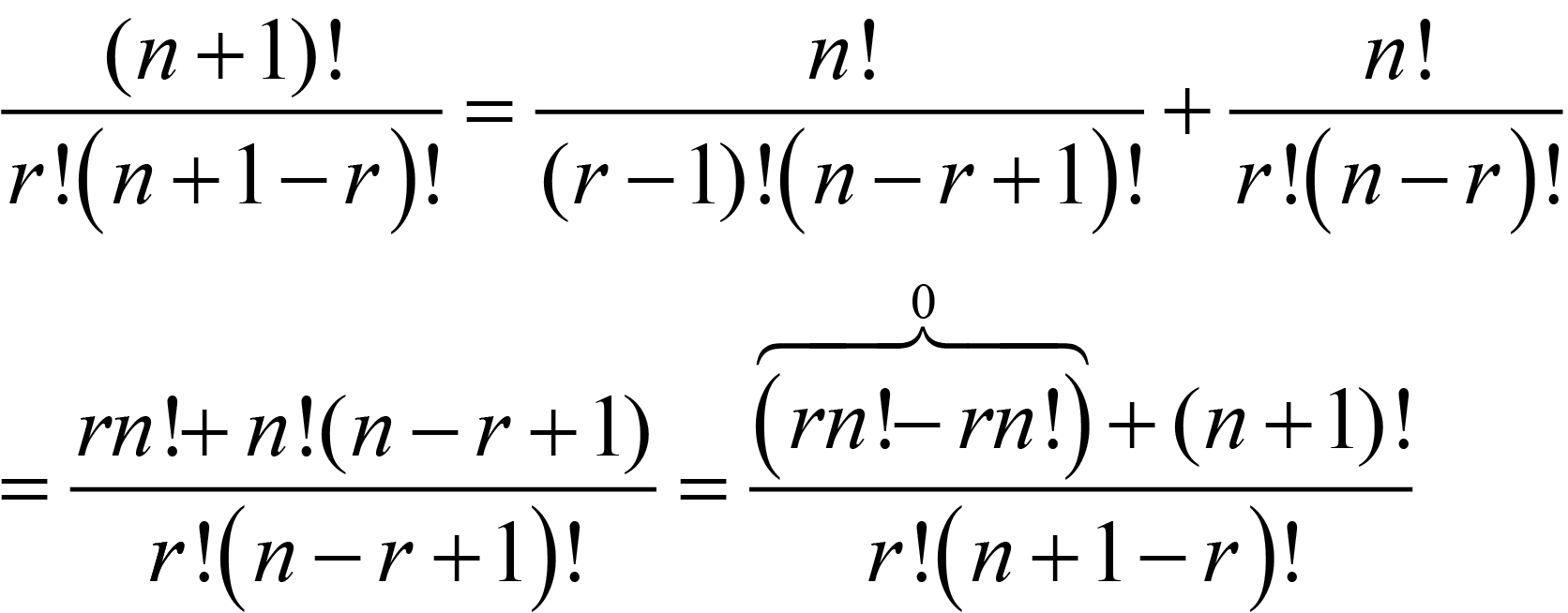None:
Polyps:
Strongs:Three Kings From Ten

The number of ways a collection of n objects can be split into a collection of r objects is given by the formula:(1.1)

Of course, this is readily stated as the number of ways nCr to choose r objects from a collection of n objects.

Yet where does this come from? We simply have to rearrange the formula to form the statement where the number of ways to choose n objects in a specific order is n!. (I.e there are n choices for the first object, n-1 for the second, n-2 for the third, right down to a single choice for the last object in the collection.) So, n! = n(n-1)(n-2)...3*2*1.

Then simply, we have to state that the number of ways (n! in total) to arrange n objects is the same as some X which is the number of ways to split n elements into two sets of r and n-r, multiplied by the number of ways to arrange the two groups remaining.

In order for this to make sense, If we split the group into two sets, of r objects and n-r objects in X number of ways then n! must be the product of X with n!(n-r)! by induction.

Then simply rearranging we end up with X = nCr the formula (1.1) as above.

Of course, it must be shown that no arrangement belongs in two different "splits". I.e. that we have not counted any arrangement more than once.

As every split is different in that at least one object must be added to the set of r from the set of n-r and another removed from the collection of r to the complement collection of n-r, there is always at least one single object in each collection which is not present in any other collection of r chosen from the n total objects. This is because the order of objects matters, and when counting the r! and (n-r)! arrangements there will be, when each partition is aligned and compared; (each having a subset of r elements unique to that "split" and which despite there being every arrangement of those r elements taken into account); no equality: as the sets upon which the count is made are different, no two arrangements share the same collection of objects and therefore not the same permutations, so the formula satisfies the condition of uniqueness posed above.

On induction we have;(1.2)

So, this may be familiar to you from Pascal's triangle where every entry is the sum of the two entries above it in the triangle. This may be restated as the number of way to choose r objects from n+1 objects is the number of ways to choose r from n objects added to the number of ways to choose r-1 objects from n objects. Why?

If we have n+1 objects and we choose a subset of r objects, choosing one object T of the whole collection in particular, T either belongs to the subset of r objects or not. If T so belongs, we may calculate the arrangements in n objects of the remaining r-1 elements with nC(r-1), otherwise if the object T does not belong to the r chosen elements we may choose the r elements from n in nCr ways. Since these are the only two scenarios for arranging a particular object of the n+1, we have (n+1)Cr = nCr + nC(r-1) as in equation (1.2).

So, as from the book, the number of ways to choose three kings from ten is 10!/(3!*7!) = 10*9*8/(1*2*3) = 120. Also, the number of ways to choose three elements from seven is 7!/(3!*4!) = 7*6*5/(1*2*3) = 35.

I hope that there is no longer any mystery over the inclusion of these significant numbers.